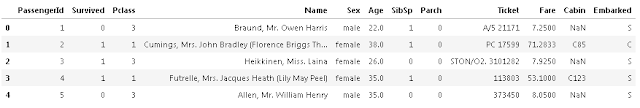# Logistic Regression :

Welcome everyone, this is first post of LOGISTIC REGRESSION. In the previous post we see Linear regression.
If you are unknown about  the Linear regression then CLICK HERE.
OK Let’s start :
For this post we will  working with the Titanic Data Set. This is a very famous data sets and very often is a student first step in machine learning section.
We will  be try to predict a classifications- survival or deceasd. Let’s begin our understanding of implementing Logistic Regression in Python for classifications.
We will  use a “semi-clean” version of the titanic data set, if you use the data set hosted directly on Kaggles, you may need to do some additional cleaning not shown in this lecture notebooks.

## Import Libraries

Let’s import some library to get start:s
`import pandas as pdimport numpy as npimport matplotlib.pyplot as pltimport seaborn as sns%matplotlib inline`

## The Data

Let’s start by reading in the titanic_trains.csv files into a pandas dataframes.
`train = pd.read_csv('titanic_trains.csv')`
`train.head()`fig 01) Logistic Regression-plot and explained Algorithms

# Exploratory Data Analysis:

Let’s begin some exploratory data analysis! We will start by checking out missing data.

## Missing Data

We can use seaborn to create a simple heatmap to see where we are missing datas .
`sns.heatmap(trains.isnull(),yticklabels=False,cbar=False,cmap='viridis')`
`<matplotlib.axes._subplots.AxesSubplot at 0x11a56f7b8>`Roughly 30 percent of the Ages data is missing. The proportion of Ages missing is likely small enough for reasonable replacement with some form . Looking at the Cabin columns, it look like we are just missing too much of that data to do something useful with at a basic levels. We will probably drop this laters.
Let’s continue on by visualizing some more of the data, Check out the graph for full explanations over these plots.

`sns.set_style('whitegrid')sns.countplot(x='Surviveds',data=train,palette='RdBu_r')`
`<matplotlib.axes._subplots.AxesSubplot at 0x11afae630>``sns.set_style('whitegrids')sns.countplot(x='Surviveds',hue='Sex',data=trains,palette='RdBu_r')`
`<matplotlib.axes._subplots.AxesSubplot at 0x11b004a20>``sns.set_style('whitegrids')sns.countplot(x='Surviveds',hue='Pclass',data=trains,palette='rainbow')`
`<matplotlib.axes._subplots.AxesSubplot at 0x11b130f28>``sns.distplot(train['Ages'].dropna(),kde=False,color='darkred',bins=40)`
`<matplotlib.axes._subplots.AxesSubplot at 0x11c16f710>``train['Ages'].hist(bins=40,color='darkred',alpha=0.8)`
`<matplotlib.axes._subplots.AxesSubplot at 0x11b127ef0>``sns.countplot(x='SibSp',data=trains)`
`<matplotlib.axes._subplots.AxesSubplot at 0x11c4139e8>``train['Fare'].hist(color='green',bins=40,figsize=(9,4))`
`<matplotlib.axes._subplots.AxesSubplot at 0x113893048>`IN THE NEXT POST WE WILL SEE CUFFLINKS FOR PLOT
for similar kin of post click here

### (If you like post then please like and share)

BEST OF LUCK!!!!

### sachin Pagar

I am Mr. Sachin pagar Embedded software engineer, the founder of Sach Educational Support(Pythonslearning), a Passionate Educational Blogger and Author, who love to share the informative content on educational resources.